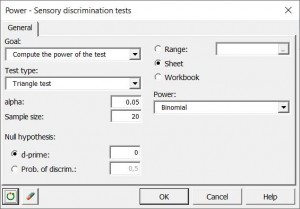# Power for Sensory discrimination tests

This tool allows you to control the power or number of subjects in sensory discrimination tests. The discrimination tests available in XLSTAT are the triangle, duo-trio, two-of-five, 2-AFC, 3-AFC and tetrads tests.## Why should we compute the power of sensory discrimination tests?

Experimental planning is a fundamental step for anyone who wants to ensure that the collected data will be usable under the best possible statistical conditions. There is no point in having products evaluated by a panel of subjects if they cannot then be compared under satisfactory statistical conditions.

XLSTAT Power for Sensory discrimination tests aims to provide sensory analysis specialists with a simple and powerful tool prior to the implementation of a sensory discrimination test in order to evaluate a set of products.

Using this tool, you will be able to:

• determine the power according to the number of subjects
• determine the number of subjects according to the power

When testing a hypothesis using a statistical test, there are several decisions to take:

• The null hypothesis H0 and the alternative hypothesis Ha.
• The statistical test to use.
• The type I error also known as alpha. It occurs when one rejects the null hypothesis when it is true. It is set a priori for each test and is 5%.

The type II error or beta is less studied but is of great importance. In fact, it represents the probability that one does not reject the null hypothesis when it is false. We cannot fix it up front, but based on other parameters of the model we can try to minimize it. The power of a test is calculated as 1-beta1−beta and represents the probability that we reject the null hypothesis when it is false.

We, therefore, wish to maximize the power of the test. XLSTAT computes the power when other parameters are known. For a given power, it also allows calculating the sample size (number of subjects) that is necessary to reach that power.

For more details on sensory discrimination tests, please refer to the Designs for sensory discrimination tests as well as the Sensory discrimination tests.

## How to configure the power calculator for the sensory discrimination tests?

You will find below the description of the various elements of the dialog box.

Goal: Choose between computing power and sample size estimation.

Test: Select the test you want to apply.

Alpha: Enter the value of the type I error.

Power (when sample size estimation has been selected): Enter the value of the power to be reached.

Sample size (when power computation has been selected): Enter the sample size (number of subjects).

Null hypothesis:

D-prime: Activate this option if you want to use a fixed value for d′. Then enter the value in the available textbox. The null hypothesis of the test is then "d′ is equal to x", with x the chosen value.

pD: Activate this option if you want to use a fixed value for the probability of discrimination. Then enter the value in the available textbox. The null hypothesis of the test is then "The probability of discrimination is equal to x", with x the chosen value.

Power: Select the distribution to be used to compute the power.

## What are the results of the power calculator for sensory discrimination tests?

A table of results is displayed. It is composed of 2 columns: the proportion of correct answers, followed by the sample size (or power, depending on the parameters selected in the dialog box). It is used to construct the simulation graph displayed below the table.### analyze your data with xlstat

14-day free trial

Included in

Related features